# Number of Days Between Dates in Excel & Google Sheets

This tutorial will teach you how to calculate the number of days between two dates in Excel and Google Sheets.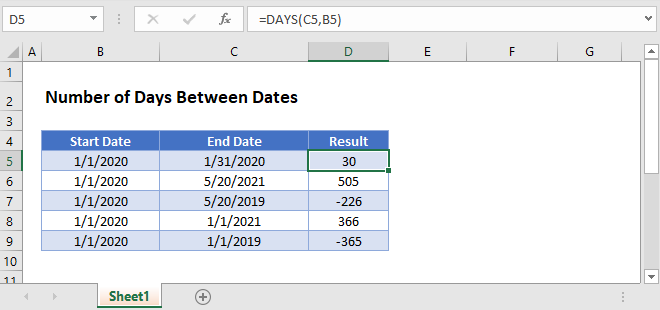## Excel Subtract Dates

In Excel, dates are stored as serial numbers: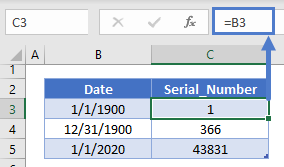This allows you to subtract dates from one another to calculate the number of days between them:

``=C3-B3``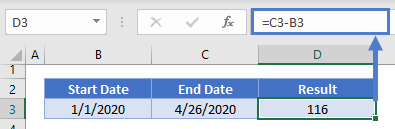If the End Date is before the Start Date you’ll receive a negative answer: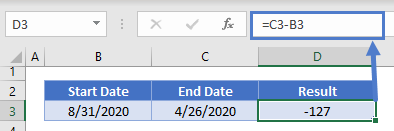If you need the absolute number of days between the dates, use the ABS Function to return the absolute value:

``=ABS(C3-B3)``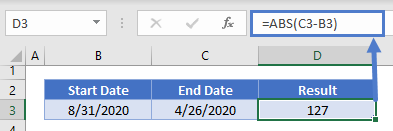### Subtract Dates with Times

In Excel, times are stored as decimal values. A decimal value attached to a serial number represents a Date & Time: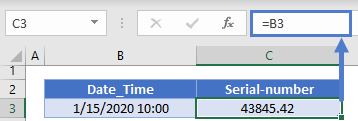If you subtract a Date and Time from another Date and Time. You’ll receive a Date and Time answer (number of days, hours, minutes, seconds between the two dates):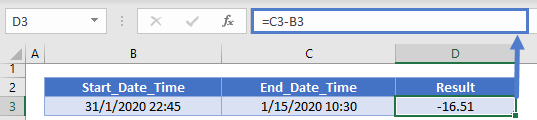Notice how the number of days between the dates is *3*, but the decimal value is *2.2* because of the time difference?  This may or may not be what you want.

Instead, you could use the TRUNC Function to find the difference between the dates:

``=TRUNC(C3)-TRUNC(B3)``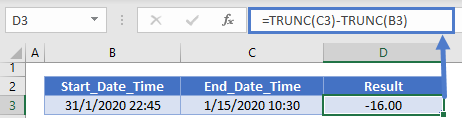But you can also use the DAYS or DATEDIF functions for an easier calculation…

## DAYS Function – Calculate Difference Between Dates

The DAYS Function calculates the number of days between dates, ignoring times.

``=DAYS(C3,B3)``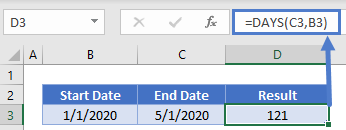The DAYS Function will return negative values, so you may want to use the ABS Function for the absolute number of days between dates:

``=ABS(DAYS(C3,B3))``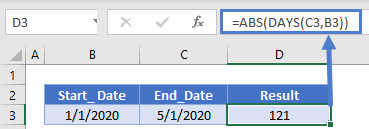### DAYS360 Function

The DAYS360 Function works the same as the DAYS Function, except it assumes a 360-day year where each month has 30 days. Notice the difference in calculations:

``=DAYS360(B3,C3)``

###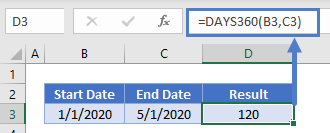DATEDIF Function – Number of Days Between Dates

The DATEDIF Function can be used to calculate the date difference in various units of measurement, including days, weeks, months, and years.

To use the DATEDIF Function to calculate the number of days between dates set the unit of measurement to “d” for days:

``=DATEDIF(B3,C3,"d")``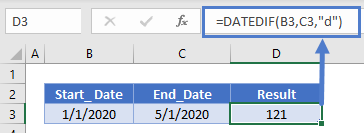Unlike the other methods, the DATEDIF Function will not work if the end_date is before the start_date, instead it will throw a #NUM! error.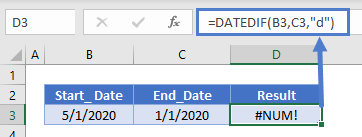To calculate difference between dates with other units of measurement use this table as a reference: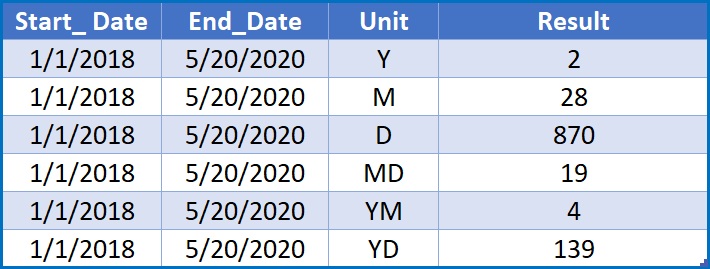## Calculate Number of Days Between Today and Another Date

To calculate the number of days from Today to another date, use the same logic with the TODAY Function for one of the dates.

This will calculate the number of days since a date:

``=DAYS(TODAY(),B3)``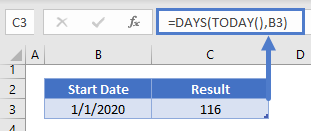This will calculate the number of days until a date:

``=DAYS(B3,TODAY())``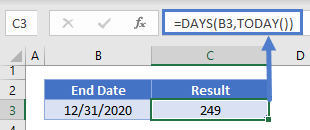### Easy Excel Automation## Calculate Working (Business) Days Between Dates

The NETWORKDAYS Function allows you to calculate the number of working (business) days between two dates:

``=NETWORKDAYS(B4,C4)``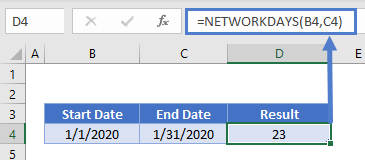By default, NETWORKDAYS will ignore all holidays. However you can use a 3rd optional argument to define a range of holidays:

``=NETWORKDAYS(B4,C4,F3:F4)``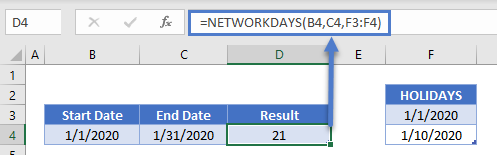## Google Sheets – Days Between Dates

All of the above examples work exactly the same in Google Sheets as in Excel.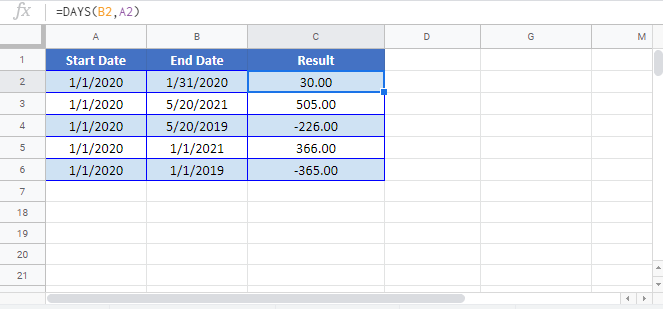### Excel Practice Worksheet

Practice Excel functions and formulas with our 100% free practice worksheets!